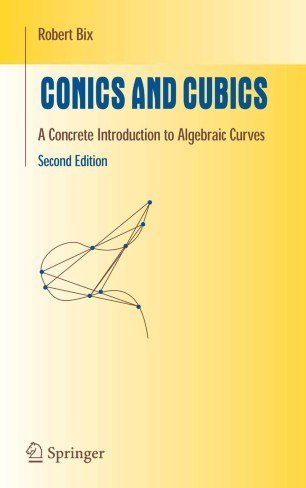# Conics and Cubics

## A Concrete Introduction to Algebraic Curves

• Robert BixTextbook

Part of the Undergraduate Texts in Mathematics book series (UTM)

1. Front Matter
Pages i-viii
2. Pages 1-68
3. Pages 69-126
4. Pages 127-244
5. Pages 245-339
6. Back Matter
Pages 340-347

### Introduction

Conics and Cubics is an accessible introduction to algebraic curves. Its focus on curves of degree at most three keeps results tangible and proofs transparent. Theorems follow naturally from high school algebra and two key ideas: homogenous coordinates and intersection multiplicities.

By classifying irreducible cubics over the real numbers and proving that their points form Abelian groups, the book gives readers easy access to the study of elliptic curves. It includes a simple proof of Bezout's Theorem on the number of intersections of two curves.

The book is a text for a one-semester course on algebraic curves for junior-senior mathematics majors. The only prerequisite is first-year calculus.

The new edition introduces the deeper study of curves through parametrization by power series. Two uses of parametrizations are presented: counting multiple intersections of curves and proving the duality of curves and their envelopes.

"The book...belongs in the admirable tradition of laying the foundations of a difficult and potentially abstract subject by means of concrete and accessible examples."

- Peter Giblin, MathSciNet

### Keywords

Area Grad algebra algebraic curve calculus geometry mathematics proof theorem

#### Authors and affiliations

• Robert Bix
• 1
1. 1.Department of MathematicsThe University of Michigan-FlintFlint

### Bibliographic information

• DOI https://doi.org/10.1007/0-387-39273-4